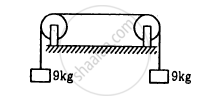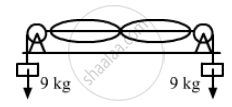Department of Pre-University Education, KarnatakaPUC Karnataka Science Class 11
Advertisement Remove all ads

# The length of the wire shown in figure (15-E8) between the pulley is 1⋅5 m and its mass is 12⋅0 g. Find the frequency of vibration with which the wire vibrates in two - Physics

Sum

The length of the wire shown in figure between the pulley is 1⋅5 m and its mass is 12⋅0 g. Find the frequency of vibration with which the wire vibrates in two loops leaving the middle point of the wire between the pulleys at rest.Advertisement Remove all ads

#### Solution

Given:
Length of the wire between two pulleys (L) = 1.5 m
Mass of the wire = 12 gm

$\text{ Mass per unit length,} m = \frac{12}{1 . 5} g/m$

$= 8 \times {10}^{- 3} kg/m$

$Tension in the wire, T = 9 \times g$

$= 90 \text{ N }$Fundamental frequency is given by:
$f_0 = \frac{1}{2L} \sqrt{\left( \frac{T}{m} \right)}$
For second harmonic (when two loops are produced):

$f_1 = 2 f_0 = \frac{1}{1 . 5} \sqrt{\left( \frac{90}{8} \times {10}^{- 3} \right)}$

$= \frac{\left( 106 . 06 \right)}{1 . 5}$

$= 70 . 7 Hz \approx 70 Hz$

Concept: Wave Motion
Is there an error in this question or solution?
Advertisement Remove all ads

#### APPEARS IN

HC Verma Class 11, 12 Concepts of Physics 1
Chapter 15 Wave Motion and Waves on a String
Q 39 | Page 326
Advertisement Remove all ads
Advertisement Remove all ads
Share
Notifications

View all notifications

Forgot password?